Lee, C.-G., Kim, M.-J., and Eom, C.-D. (2022). "Kinetic study of torrefied woody biomass via TGA using a single heating rate and the model-fitting method," BioResources 17(1), 411-428.

#### Abstract

A model-fitting method at a single heating rate (10 °C·min-1) was used to investigate the thermal kinetic characteristics of torrefied woody biomass. The kinetic parameters were examined for pine, oak, and bamboo samples with the order of the reaction set ranging from 0.1 to 0.5 and 1.0. Based on the thermogravimetric, derivative thermogravimetric, and derivative2 thermogravimetric curves obtained, the ranges at which substantial hemicellulose and cellulose pyrolysis occurs were set as the analysis range, and the kinetic parameters of each species were analyzed. The activation energy and pre-exponential factor were obtained at these analytical ranges using two differential methods (Friedman and Chatterjee-Conard) and an integral method (Coats-Redfern). Although there were numerical differences between the results of the differential and integral methods, the thermal properties of each sample exhibited a consistent trend. Softwood was found to have the highest reactivity and intermolecular collisions per unit weight during thermal decomposition. In the case of the torrefied oak and torrefied bamboo, considering that the carbon content and fixed carbon content were approximately 24% to 25% higher than the softwood, it is appropriate to consider the thermal characteristics of each species for producing a solid fuel based on the application.

Kinetic Study of Torrefied Woody Biomass via TGA Using a Single Heating Rate and the Model-Fitting Method

Chang-Goo Lee, Min-Ji Kim, and Chang-Deuk Eom *

A model-fitting method at a single heating rate (10 °C·min-1) was used to investigate the thermal kinetic characteristics of torrefied woody biomass. The kinetic parameters were examined for pine, oak, and bamboo samples with the order of the reaction set ranging from 0.1 to 0.5 and 1.0. Based on the thermogravimetric, derivative thermogravimetric, and derivative2 thermogravimetric curves obtained, the ranges at which substantial hemicellulose and cellulose pyrolysis occurs were set as the analysis range, and the kinetic parameters of each species were analyzed. The activation energy and pre-exponential factor were obtained at these analytical ranges using two differential methods (Friedman and Chatterjee-Conard) and an integral method (Coats-Redfern). Although there were numerical differences between the results of the differential and integral methods, the thermal properties of each sample exhibited a consistent trend. Softwood was found to have the highest reactivity and intermolecular collisions per unit weight during thermal decomposition. In the case of the torrefied oak and torrefied bamboo, considering that the carbon content and fixed carbon content were approximately 24% to 25% higher than the softwood, it is appropriate to consider the thermal characteristics of each species for producing a solid fuel based on the application.

DOI: 10.15376/biores.17.1.411-428

Keywords: Torrefied biomass; Kinetics; Single heating rate; Differential and integral methods; Activation energy; Pre-exponential factor

Contact information: Division of Wood Industry, Department of Forest Products and Industry, National Institute of Forest Science, Seoul 02455 Republic of Korea; * Corresponding author: willyeom@korea.kr

INTRODUCTION

Research on pretreatments to improve the energy density of biomass has been steadily progressing. Torrefaction, a mild pyrolysis technology, is a pretreatment technique for improving biomass feedstock to be in the same league as coal by its treatment within a reaction temperature range of 200 to 300 °C in an anoxic atmosphere (Stelt et al. 2011; Mamvura and Danha 2020). Over a 10-year period, torrefied wood has been introduced as a high-efficiency fuel along with information on its chemical composition (Prins et al. 2006; Phanphanich and Mani 2011; Bach and Tran 2015; Chen et al. 2018, 2021). As knowledge on the thermal decomposition of biomass is critical for investigating its thermal properties with respects to biomass energy utilization, information on the chemical kinetics of biomass thermal decomposition and the investigation of its reaction mechanism are crucial. Thermogravimetric analysis (TGA), which is commonly used for analyzing the kinetics in a pyrolysis reaction of polymer materials, measures the mass loss of a sample as a function of the temperature. Kinetic analysis using TGA can be performed under isothermal and nonisothermal conditions, which can also be divided into model-free and model-fitting methods (Vyazovkin and Wight 1999). In the model-free method, the activation energy can be calculated at multiple heating rates without any assumptions on the reaction model (Rana et al. 2013). On the other hand, the advantage of this method is that the area can be set for kinetic analysis from the results from TG and derivative TG (DTG), which are obtained from the model-fitting method under a condition of single heating rate. Therefore, the reaction model can be assumed, and the parameters of the reaction kinetics are arbitrarily determined from the thermogravimetric (TG) and derivative TG (DTG) curves at a single heating rate (Jang et al. 2020). Studies on the reaction kinetics of woody biomass, i.e., lignocellulosic biomass, have evaluated its fuel efficiency as a solid fuel (Idris et al. 2010; Chen et al. 2012; El-Sayed and Mostafa 2014, 2015; Saldarriaga et al. 2015; Carrier et al. 2016). In particular, certain reaction kinetic studies on torrefied woody biomass have reported that the activation energy decreases with increases in the treatment temperature and residence time of torrefaction (Ren et al. 2013; Gaitán-Álvarez et al. 2018; Barzegar et al. 2020). However, in most previous studies, multiple heating rates have primarily been used and the order of the reaction has been arbitrarily set using the model-free method (Wang et al. 2018). Although it is beneficial to investigate the overall thermal degradation behavior of the sample through the change in the heating rate using the model-free method, investigation of the thermal degradation behavior of the major components, i.e., cellulose, hemicellulose, lignins, etc., of a biomass sample using the model-fitting method at a single heating rate can provide useful data. More specifically, in torrefied wood, the proportion of hemicellulose is very low due to thermal decomposition, and thus, low-concentration hemicellulose is thermally decomposed relatively quickly and converted in the temperature range of thermal decomposition generally assigned to cellulose. It is important to select the proportions of cellulose and hemicellulose in each temperature range of thermal decomposition giving the best response under various conditions of the heating rates. In this study, a kinetic analysis was employed in an effort to demonstrate changes associated with the wood torrefaction process. Focus was placed on the fact that torrefied wood has low hemicellulose proportions in the wood cell wall, and this aspect can be quantified via a model-fitting method at the single heating rate.

Therefore, the final objective of this study was to clarify the pyrolysis mechanism of torrefied wood through its kinetics. In addition, a comparative analysis was performed between the equations of the differential and integral kinetics. In this study, the authors focus on the pyrolysis mechanism of cellulose and hemicellulose, while excluding lignins. Lignins, which are a primary component in the wood cell wall, continuously degrade under a wide temperature range from the beginning of pyrolysis to the end. Therefore, the evaluation of the wide range of thermal decomposition temperatures for lignins at a single heating rate is difficult. Hence, the range for lignins was not included in this study because the thermal decomposition parameters cannot be accurately determined.

EXPERIMENTAL

Materials

Samples of Pinus densiflora Siebold & Zucc, Quercus serrata Thumb. ex Murray, and Phyllostachys nigra var. Henosis honda (later referred to as pine, oak, and bamboo, respectively) were used in this study. The pine and oak samples included wood chips for pulp, and the bamboo was cut to a length of approximately 30 mm. The torrefaction treatment of the samples was performed using a self-made apparatus (also known as a “wood roaster”) as described in a previous study by Lee et al. (2016). The apparatus was rotated a speed of 45 rpm, while blocking oxygen from the outside. When the internal temperature of the roaster reached 220 °C through the application of direct heat at the bottom of the roaster, the top was opened, and the wooden chip samples were inserted. After 300 s in the roaster, the torrefied materials were removed and crushed. In addition, the torrefied wood powder of each species, including the untreated materials, were passed through a #40 standard sieve, and these samples were used as the experimental material. In this study, the following abbreviations are used: for the untreated samples – untreated pine (UP), untreated oak (UO), and untreated bamboo (UB); for the torrefied samples – torrefied pine (TP), torrefied oak (TO), and torrefied bamboo (TB).

Methods

Elemental analysis

The elemental analysis was carried out through an elemental analyzer (EA 1108-CNHS-O, FISON Instruments, USA). All samples were instantaneously combusted in a pure oxygen atmosphere for oxidation. A quantitative analysis was carried out on C, H, N, S among the samples using a thermal conductivity detector, and O was generated by pyrolysis, transformed into CO, and redetected again through the thermal conductivity detector. The measurement was carried out three times repeatedly, and one-way analysis of variance (ANOVA) data evaluation was carried out using Duncan’s new multiple range test to compare torrefied samples and untreated samples in different groups.

Ultimate analysis

The ultimate analysis was carried out through an ultimate analyzer (CKIK 5E-MACIV, Changsha Kaiyuan Instrument Co., China). The experimental conditions were to measure the moisture content at 105 °C for 1 hour. Then, it was retained for 1800 s to 750 °C for 1 h. The ash content was measured for 2 h. Lastly, the temperature was raised to 950 °C, at which temperature the volatile content was measured during 420 s. At this time, the fixed carbon content was mathematically calculated by subtracting moisture, ash, and volatile matter from the weight of the first state of each sample. The measurement and evaluation was repeated three times. Also, a one-way analysis of variance (ANOVA) data evaluation was done using Duncan’s new multiple range test to compare torrefied samples and untreated samples as measured in two groups.

Thermogravimetric analysis (TGA)

Thermogravimetric analysis was performed using a TGA/DSC1 thermogravimetric analyzer (Mettler-Toledo, Greifensee, Switzerland). The gas used N2 with a flow rate of 50 mL·min-1. The temperature increase was set to 10 °C·min-1 as a single heating rate within a temperature range of 30 to 800 °C.

Kinetic studies

Based on this theory, the basic kinetics model using TGA is generally represented by , which is the change in the conversion rate with time at a constant temperature. Here, X is the conversion rate depending on the thermal decomposition of the sample, as expressed in Eq. 1,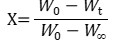(1)

where W0 (g) is the initial sample weight, Wt (g) is the instantaneous sample weight, and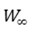(g) is the final sample weight. The change in the conversion rate can be expressed as a function f(X) at a constant temperature. This is the most extensively used function in polymer kinetics in which solid materials are decomposed into gaseous products. In addition, this can be expressed as Eq. 2,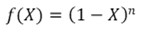(2)

where n is the order of the reaction. If pyrolysis is the nth reaction, the temporal change of the conversion rate has a proportional relationship with the constant rate k, and the following equation can be established, as shown in Eq. 3,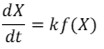(3)

where the constant rate k depends on the temperature, and a commonly used flammable substance under nonisothermal conditions can be expressed as follows using Arrhenius’s formula, as shown in Eq. 4,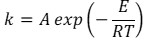(4)

where E (kJ∙mol-1) is activation energy, A (s-1) is a pre-exponential factor, R (J∙K-1∙mol-1) is the universal gas constant, and T is the absolute temperature.

Finally, combining Eqs. 3 and 4 yields Eq. 5: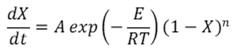(5)

In this study, based on Eq. 5, the differential and integral methods were used, before and after the torrefaction of the samples of each species, to obtain the parameters of the reaction kinetics.

Differential methods

Two analysis methods were used to obtain the parameters of the reaction kinetics in the differential method. Friedman’s formula, which is commonly used in the thermal decomposition of solid materials, was first adopted. However, this method should be applied under multiple heating rates for a fixed conversion rate. The application of a single heating rate as a condition is not preferred. As such, in order to accurately obtain the velocity variable, it is necessary to compare the tendency fluctuation with the control group. Therefore, in the Friedman method, n = 1 was assumed and the obtained parameters were used as comparative data in this study. In addition, the analysis was carried out from the viewpoint of torrefied samples versus untreated samples and the difference between tree species among the torrefied samples. Friedman’s formula can be expressed as follows, using the algebraic expression from Eq. 5 to yield Eq. 6,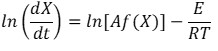(6)

(Friedman 1965; Sbirrazzuoli 2007; Sbirrazzuoli 2020).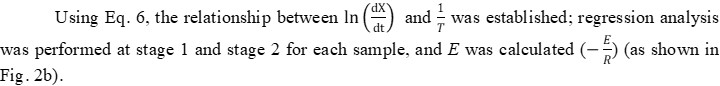Furthermore, the Chatterjee-Conard formula, another differential method, was adopted to calculate each parameter, substituting an arbitrary order of the reaction based on Friedman’s formula with the n = 1 condition (Chatterjee and Conard 1968). Generally, it is preferable to select the order of the reaction that achieves maximum linearity when obtaining the parameters of the reaction kinetics. Moreover, it has been reported that the most suitable order of the reaction under high temperature conditions is generally less than 0.5 (Mitchell 1989; Hurt and Calo 2001; Murphy and Shaddix 2006; Murphy and Shaddix 2010). Therefore, the order of the reaction was assumed to be n = 0.1 to 0.5, and n = 1.0 was included in the comparative analysis in Friedman’s formula. However, it was assumed that it follows two different order of reactions (Friedman’s formula and Chatterjee-Conard’s formula) simultaneously for some different reactions under arbitrarily selecting the order of reactions, respectively. Parameter A can be calculated based on ln(A) on the regression line segment (Fig. 1b) or calculated directly using exponential functions (Aktar and Adal 2019). In this study, A was obtained directly using Arrhenius’s formula.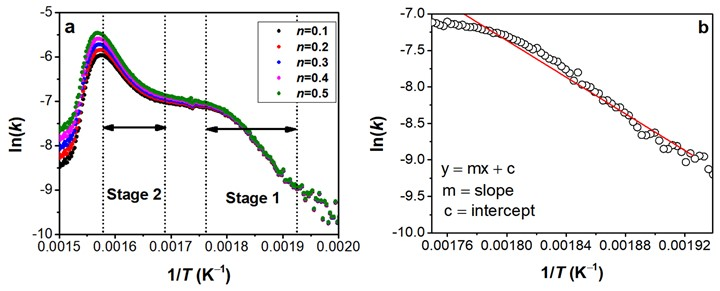Fig. 1. Kinetic curves simulated according to the selected kinetic equation: (a) example of the kinetic parameters and analysis range for each order of the reaction; and (b) example of the kinetic parameter obtained through regression line analysis

Integral methods

For obtaining the parameters of the reaction kinetics using the integral method, the Coats-Redfern formula, which is often used in the model-fitting method and extensively used in the thermal decomposition of solid substances, was adopted (Coats and Redfern 1964). Substituting the constant heating rate applied in TGA (as shown in Eq. 5), resulted in Eq. 7,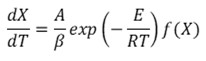(7)

When analyzed under nonisothermal conditions, the constant heating rate can be expressed as Eq. 8,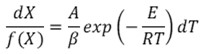(8)

On integrating Eq. 8, the following equation can be obtained, as shown in Eq. 9,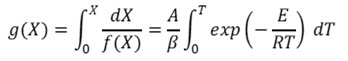(9)

which in this case, g(X) is an integral type of reaction model.

In addition, with when and when , integration is again performed to obtain Eq. 10,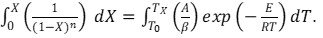(10)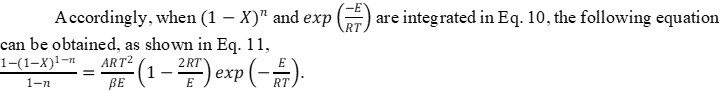(11)

which, when considered as a logarithmic expression, is expressed as Eq. 12,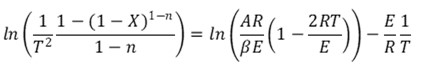(12)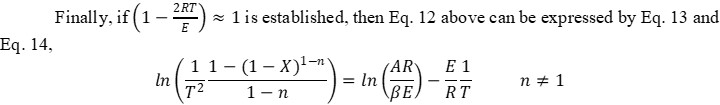(13)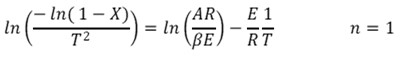(14)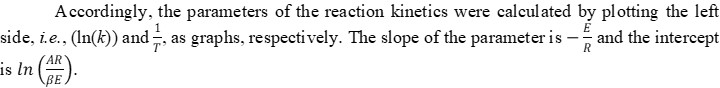Therefore, E and A can be calculated. However, for n ≠ 1, which is the condition shown in Eq. 13, n was substituted assuming a range of 0.1 to 0.5 in the same manner as in the differential method described in the previous section. For n = 1, the calculation was performed using Eq. 14.

RESULTS AND DISCUSSION

Sample Characteristics and Chemical Compositions

Tables 1 and 2 show the elemental and chemical composition results of each specimen. In addition, as the experiments were repeated three times, statistical testing was performed to determine the significant differences among the six specimens, i.e., the torrefied samples versus the untreated samples, through Duncan’s new multiple range test. Based on the statistical results, it was confirmed that the increase in carbon elements and fixed carbon, which characterizes torrefied wood, was significantly different for the torrefied and untreated samples (at a 99% significance). Thus, the authors attempted to determine the effect of torrefaction on woody biomass through the reaction kinetics under a single heating rate based on the premise that the chemical properties of each sample were significantly different.

Table 1. Analysis Results for Each Specimen via Proximate Analysis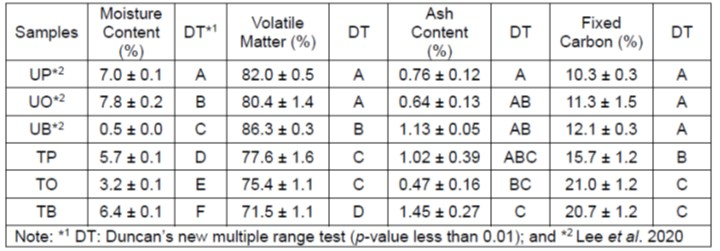Table 2. Analysis Results for Each Specimen via Ultimate Analysis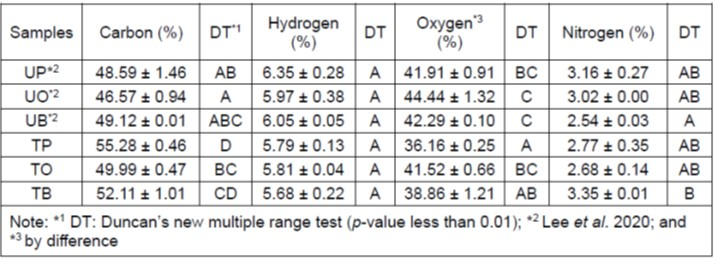Analysis Range Selection based on TG, DTG, and D2TG Curves

Figure 2 displays the TG reduction rate as well as the DTG and D2TG curves. For a comparative analysis of the reaction kinetics between the torrefied and untreated samples, an analysis range was set for each sample. For example, Figs. 2a and 2b depict the parameters of oak. In the TG curve shown in Fig. 2a, it can be observed that the hemicellulose and cellulose are thermally decomposed at temperature ranges of 245 to 290 °C and 320 to 360 °C, respectively. In addition, the DTG and D2TG curves indicated the pyrolysis of hemicellulose. The decomposition of hemicellulose can also be clearly identified through the shoulder in the DTG and D2TG curves. Here, the regions where the hemicellulose and cellulose substantially thermally decompose are denoted as “stage 1” and “stage 2,” respectively, and they were set as the analysis ranges to study the reaction kinetics.

However, as shown in Fig. 2b, the concentration of hemicellulose was considerably reduced by torrefaction, and the shoulder in graphs is not clear. Therefore, based on the section where thermal decomposition could be confirmed in the D2TG curve, the left and right sections were divided as stages 1 and 2, respectively. Even in the pine and bamboo samples, the shoulder could not be observed under torrefied conditions. In these cases, the analysis range was set based on the DTG and D2TG curves, as depicted in Fig. 2b. In addition, the information on the analysis range and the TGA results for each sample is shown in Table 3.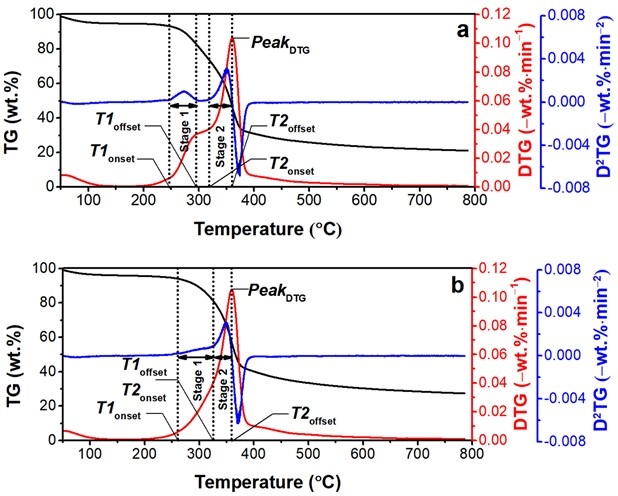Fig. 2. Examples of the analysis range selection based on TGA and the DTG and D2TG curves

Table 3. Analysis Results for Each Specimen Based on the TG and DTG Curves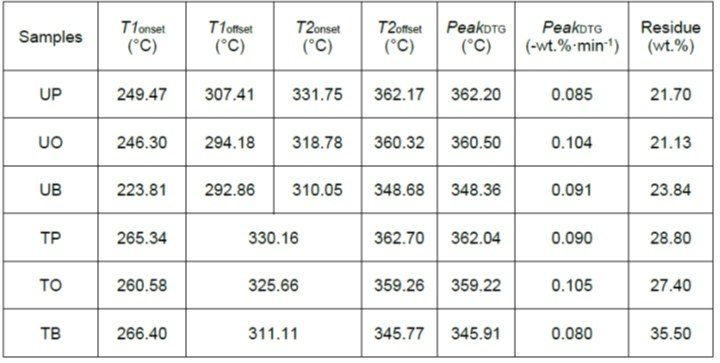Differential Methods

Figure 3 depicts the E value of each species calculated under n = 1 (order of the reaction) with Friedman’s formula. As shown in Fig. 1b, the activation energy E depends on the slope of the ln(k) parameter. Figure 3a and 3b display the results for stage 1 and stage 2, respectively. In the pyrolysis region of hemicellulose (as shown in Fig. 3a), the results of the torrefied pine and bamboo samples confirmed that there was a slight increase in the E value due to torrefaction (approximately +10%), whereas torrefied oak had lower E values, unlike the other species (-20.2%). This may be due to the higher hemicellulose content of hardwood compared to softwood and bamboo; for instance, in untreated oak hardwood, the necessary activation energy for conversion to cellulose is higher than in the untreated pine and bamboo samples (Kim et al. 2007).

In the pyrolysis region of cellulose (Fig. 3b), the E values of the torrefied oak samples were approximately 7% to 41% higher than all the other samples. Thus, it was inferred that hardwood is also affected by the thermal decomposition of cellulose because it has a relatively complex cellular structure in comparison to softwood and bamboo. Both before and after torrefaction, pine exhibited lower E values than the other species. In addition, the E value of torrefied oak was highest in the region where there is considerable cellulose thermal decomposition (+7.2% to 41.0%). Table 4 lists the values of A and R2 (coefficient of determination) calculated through Friedman’s formula. The R2 value calculated for n = 1 ranged from 0.956 to 0.999, and the correlation between the regression line and ln(k) for each sample parameter was high. In the untreated group, the A values were higher in stage 1 compared to stage 2, except for the untreated bamboo samples. This might indicate that the number of molecular collisions per time unit generated by the thermal decomposition of hemicellulose is greater than in cellulose, and the reaction rate is faster. In the torrefied group, all the samples showed higher A values in stage 2 compared to stage 1. However, in the case of hardwood, the values of the torrefied oak and untreated oak samples were considerably higher compared to the other species. This may be due to the anatomical characteristics of each species rather than the effect of torrefaction.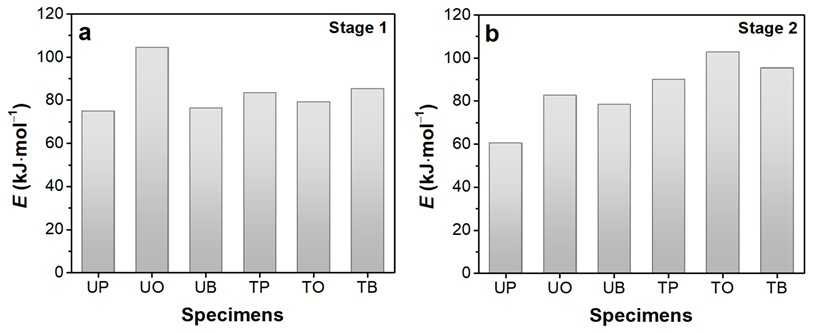Fig. 3. Results of the activation energy (E) of each sample using Friedman’s formula (n=1): (a) results in stage 1; and (b) results in stage 2

Table 4. Kinetic Parameters Extracted from the Differential Model Using the Friedman Method (n = 1.0)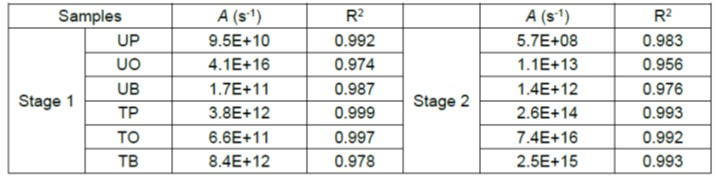Figure 4 shows the E values calculated at n = 0.1 to 0.5 and 1.0, respectively, using the Chatterjee-Conard formula, and Table 5 lists the A and R2 values. As shown in Fig. 4a, which includes the pyrolysis region of hemicellulose, the E value of the untreated pine samples was the highest for any reaction order compared to the untreated oak and untreated bamboo samples (approximately 26% to 27%) and was 18% to 23% higher than the E value of the torrefied samples.

As shown in Fig. 4b, which includes the pyrolysis region of cellulose, as the order of the reaction increased, the increase rate of the E value was higher than the rate shown in Fig. 4a, confirming that the E value of the torrefied groups was clearly higher than the E value of the untreated groups for all the species. In a study by Lee and Kim (2016), the change (decrease) in the E values of torrefied oak (treated for 300 s at 200 °C in a wood roaster) and untreated oak of the same species were approximately the same. However, the values were calculated using the model-free method at multiple heating rates, and the numerical value of the activation energy was slightly different from the one used in this study.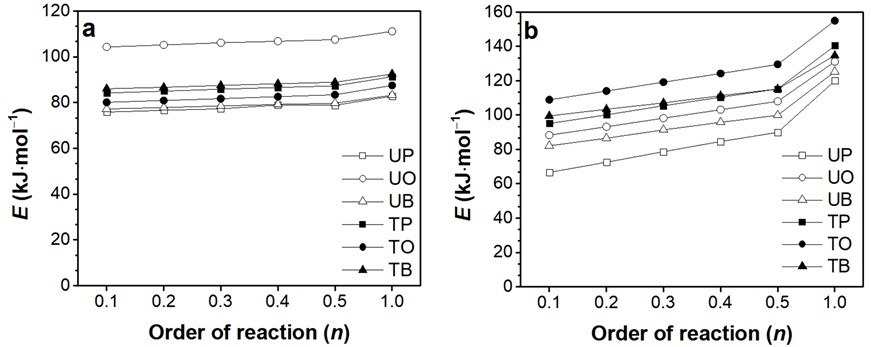Fig. 4. Results of the activation energy of each sample using Chatterjee-Conard’s method and order of the reaction condition: (a) results in stage 1; and (b) results in stage 2

Table 5. Kinetic Parameters Extracted from the Differential Model Using the Chatterjee-Conard Method